# Comparison Between 1D, 2D and 3D elements in Structural Analysis

## Introduction

Structural engineers use a great variety of members already from the conceptual phase of a building or infrastructure project. Accurate modelling of such members using finite element software, such as SkyCiv, is of great importance in the design process, as accurate modelling of the members can both reduce cost and ensure a safe design. Various modelling methods exist to simulate the structural behaviour of columns, beams, walls, or slabs using 1D, 2D and 3D elements. This article explains the main differences between the various modelling techniques used in a regular engineering design cycle. Specifically, focusing on the modelling, state of stress and deformation, and results.

## Modelling phase (pre-processing)

### Geometry

1D modelling approaches are used for modelling line-type members such as columns / piers, beams or piles. Representation of the line is self defined by the user via a section and all geometric properties of the member (width, height, etc.).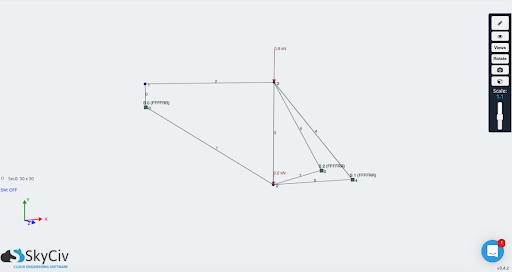2D modelling techniques are commonly used for plate-type members such as walls, slabs, shells, bridge abutments, tanks, silos or domes. For all these members, the two dimensions perpendicular to the thickness are considered as much larger than the thickness. It is therefore only the slab thickness that must be defined as section property by the user prior to the analysis. However, there exist limitations when using 2D modeling techniques as strict rules are to be adhered to, in order to obtain the most accurate results. The main rules that follows are the ratio between the thickness and area of the plate itself, as the use of a very high thickness to area ratio plate will enter the realm of 3D modelling where all assumptions made using 2D elements no longer holds true.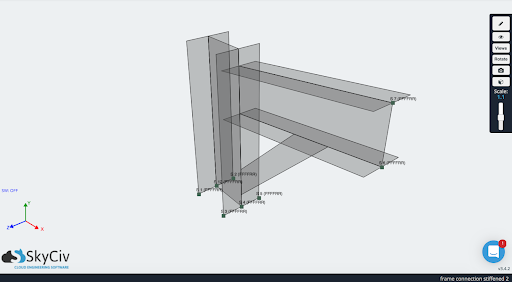3D elements can be used for modelling any structural member. In this case, each member of the model is commonly divided into equal volumetric 3D elements. As it stands, CAD programs (such as SolidWorks) are used to create these 3D models, it grants the opportunity to create more complex 3D models used for structural analysis. For instance, the same bike frame seen with the 1D elements can be replicated using 3D elements, with the following result: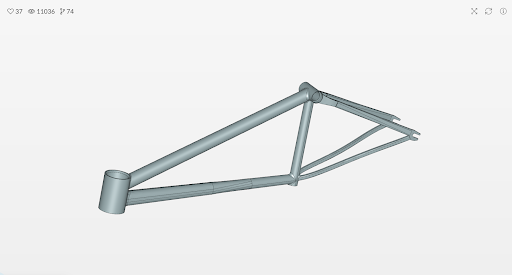Source: SimScale

A big drawback of using 3D elements, is its modelling (the above model could take up to 10x longer than 1D modelling ) and solving time. 3D elements attempts to fully capture the physics of the model, internally and externally by utilizing computation-heavy calculations required to solve the analysis at the cost of more time spent on the model itself.

### Meshing

As meshing is a crucial step in any structural analysis, it is important that users are aware of the impact that meshing plays on 1D, 2D, and 3D elements on a model.

As mentioned before, 1D elements are commonly used to represent line members and can provide accurate bending behaviour of a member. 1D element meshing is the division of the member into multiple segments, this does not affect the overall result but more segments allows smoother and better visualization of the results.

2D and 3D elements present similar traits in terms of meshing. Each member inside the model is divided into multiple parts of a certain shape, the mesh size of the model affects the final results and that the finer the mesh (smaller shapes used) the longer the time taken to solve the model. There are two shapes that are used for both 2D elements in meshing, quadrilateral and triangular elements. 3D elements shapes are variations that stem from the 2D element shapes, commonly used shapes are hexahedrons, tetrahedrons, wedges and pyramids with each offering different benefits to better model the physics of the model itself.

## Output (post-processing)

The analysis results for members modelled using 1D elements are usually given in terms of shear force and bending moment about the two main axes of the members as well as axial force and torsional moment about the axis that connects both ends of the member.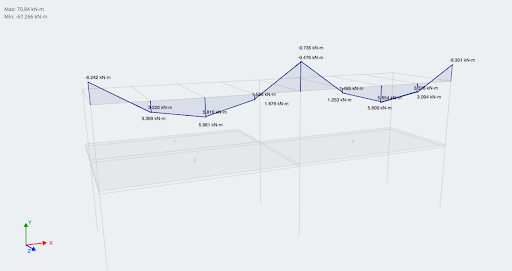Example of Bending Moment Force Diagram in 1D analysis software

For 2D elements, the output is illustrated as axial force, shear force, bending moment and torsional moment per unit length.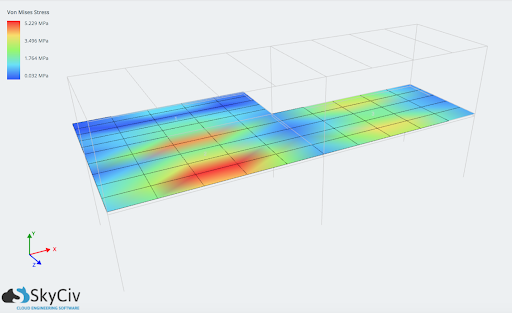Such results can include:

• Membrane forces
• Shear/moment forces in plane
• Displacements (x,y,z, sum)
• Shear (Von-mises, direct, shear, major/minor principal)
• When, brick elements are adopted, the results are given in terms of stresses. Therefore, the internal forces and support reactions of members like shear walls, shells or slabs modelled using 2D elements or brick elements is obtained through integration of internal forces/moments per unit length or stresses over the length or area of interest, respectively.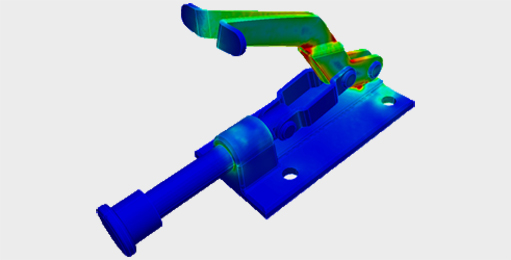Paul Comino
CTO and Co-Founder of SkyCiv
BEng Mechanical (Hons1), BCom